Zeitschriftendaten
Format
Zeitschrift
eISSN
1891-5469
Erstveröffentlichung
01 Jan 2010
Erscheinungsweise
1 Hefte pro Jahr
Sprachen
Englisch
Uneingeschränkter Zugang

# Geometric parameters optimization of planar interdigitated electrodes for bioimpedance spectroscopy

###### Eingereicht: 04 Jun 2012
Zeitschriftendaten
Format
Zeitschrift
eISSN
1891-5469
Erstveröffentlichung
01 Jan 2010
Erscheinungsweise
1 Hefte pro Jahr
Sprachen
Englisch
Introduction

Impedance spectroscopy is a powerful experimental technique that compares the electrical response of a system to a time-varying electrical excitation . This method of electrical impedance measurement has been demonstrated as a potentially useful approach in biomedical applications. This method allows the determination of physiological status of ex vivo or living tissues in addition to their electromagnetic characterization . The changes induced by some pathologies could be associated with variations of essential tissue parameters such as the physical structure or the ionic composition which can be reflected as changes in the passive electrical properties. The range of applications derived from this technique is quite wide .

Planar interdigitated electrode arrays are a commonly used electrode configuration for conductimetric sensing applications . These electrode arrays have become more prominent as a sensor device due to the ongoing miniaturization of electrodes and the low cost of these systems . An important advantage of these sensors is its simple and inexpensive mass-fabrication process and the ability to use them over a wide range of applications without significant changes in the sensor design [6, 7]. Another potential benefit is the ability to integrate electrodes with instrumentation to develop autonomous, lab-on-chip measurement systems. Typically they have been used for the detection of capacitance, dielectric constant, and bulk conductivity in biological mediums [8, 9]. We have been motivated to consider this type of geometry for biomedical applications generally and, more particularly, to measure the dielectric properties (such as conductivity and permittivity) of human blood from a droplet. A disadvantage of microelectrodes is their much higher impedances compared to macro-electrodes due to influence of interfacial capacitance. Interfacial, or double layer, capacitance arises from the interactions between ions and molecules at the interface of the electrolyte and electrode surface and has been established to be indirectly proportional to the electrode area . This double layer capacitance places a constraint on the measurement equipment and increases the measurement error in low frequencies (around 1 kHz). Consequently, microelectrode design needs to be optimized to reduce interfacial impedance as well as to extend the useful frequency probing range of the microelectrode in the lower frequency band. Two optimization rules have been proposed and theoretically studied. The first rule is the elimination of the effects of interfacial phenomena, and the second is increasing the sensitivity of microbiological measurements.

This paper presents a new approach of physical modeling system of a microelectrodes biological sensor. The correlation between design parameters and frequency behavior in a coplanar impedance sensor is presented. The theoretical optimization of the geometrical parameters of the sensor is conducted by developing impedance equations and modeling equivalent circuits. One objective was to get the optimal ratio between the width of the electrodes and the gap between the electrodes to expand the useful frequency band, and the optimal number of electrodes. A sensor model including a priori the human blood medium was described with the finite element method FEM using CoventorWare® software for simulation. The influence of the medium’s physical properties on the frequency sensor response was investigated. We simulated the influence of geometric parameters of interdigitated electrodes to define the optimal configuration for a 1mm × 1mm cross section. The results of the theoretical development of optimization of the sensor and those of the 3D modeling and simulation are discussed.

Analytical optimization of sensor
Equivalent circuit model

Figure 1.a gives the configuration of the planar structure of the interdigitated impedance sensor. Figure 1.b represents the simplified equivalent electrical circuit when such a sensor is immersed in an electrolyte. This equivalent circuit model is adapted from Hong et al.  and is also used by others [12, 13, 14, 15, 16, 17, 18]. We replaced the constant phase element (CPE), which is a measure of non-faradaic impedance arising from the interface capacitance, with a simple capacity CDL to facilitate physical modeling. In this case the CPE represents a purely capacitive impedance element corresponding to the interfacial capacitance. The electrical elements in the equivalent circuit model the physical phenomena that determine the total electrical impedance (Z) detected in the measurement cell (Figure 1.a). Thus, the equivalent model elements could be expressed in terms of physical quantities.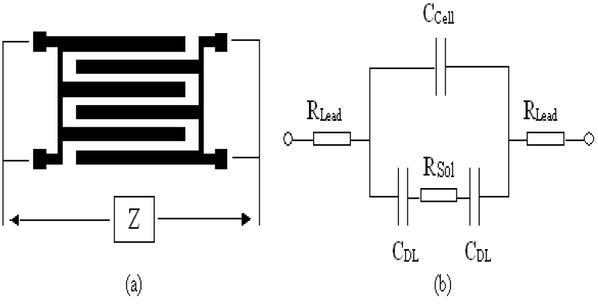(a) Configuration of interdigitated impedance cell structure and (b) its adapted equivalent circuit model. CCell and RSol models the dielectric properties of the medium under testing, and CDL models the properties of the double layer phenomena.

The resistance RSol of the medium is the sensing element and is related to the electrolyte conductivity σSol by the cell constant KCell  as described by:

$RSol =KCellσSol$$$\text{RSol =}\frac{\text{KCell}}{\text{ }\!\!\sigma\!\!\text{ Sol}}$$$KCell =2(N-1)L⋅K(k)K(1-k2)Where K(k)=∫011(1- t2)(1-k2t2)dt and k=cos(π2⋅WS + W)$\begin{align}& \,\,\,\,\,\,\,\,\,\,\,\,\,\,\,\,\,\,\,\,\,\,\,\,\,\,\,\,\,\text{KCell}\,=\frac{2}{\left( \text{N-1} \right)\text{L}}\cdot \frac{\text{K}\left( \text{k} \right)}{\text{K}\left( \sqrt{\text{1-}{{\text{k}}^{\text{2}}}} \right)} \\ & \text{Where}\,\,\text{K}\left( \text{k} \right)=\int\limits_{0}^{1}{\frac{1}{\sqrt{\left( \text{1-}\,{{\text{t}}^{\text{2}}} \right)\left( \text{1-}{{\text{k}}^{2}}{{\text{t}}^{2}} \right)}}\text{dt}}\,\,\text{and}\,\,\text{k=cos}\left( \frac{\pi }{2}\cdot \frac{\text{W}}{\text{S}\,\text{+}\,\text{W}} \right) \\ \end{align}

In the preceding equations, N is the number of fingers; S the finger spacing, W the finger width and L the finger length (see Figure 2). Figure 2 presents the electrode structure consisting of two parallel coplanar electrodes whose design (width, spacing between electrodes, and length) is periodically repeated . The function K(k) is the incomplete elliptic integral of the first kind. So, according to Olthuis et al.  the cell constant depends entirely on the geometry of the sensor. The lead resistance RLead is the result of the series resistances of the connecting wires. It was considered negligible in the physical modeling.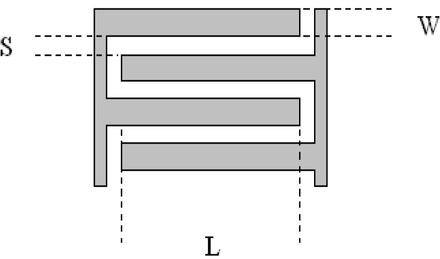Interdigitated sensor structure of 4 electrodes. The dimensional parameters L, W, and S are finger length, finger width and finger spacing, respectively.

Direct capacitive coupling between the two electrodes is represented by the cell capacitance CCell given by

$Ccell =ε 0 ⋅ ε r,SolKCell$$$\text{Ccell}\,=\frac{\varepsilon \,0\,\,\cdot \,\,\varepsilon \,\text{r,Sol}}{\text{KCell}}$$

with ε r, Sol ≈ ε r, Water ≈ 80 . CCell models the dielectric part of the medium, such as the permittivity εr,sol.

It must be noted that RSol and CCell are placed in parallel because the currents propagate both in the form of conduction currents through RSol and displacement currents through CCell.

The impedances that explain the interface phenomena at the electrode-electrolyte interfaces are simplified to the double layer capacitances, CDL. These are dependent on the electrode material and the electrolyte solution [1, 2, 3, 4, 5, 6, 7, 8, 9, 10, 11, 12, 13, 14, 15], but for horizontal electrode surfaces they can be approximated by

$CDL =0.5⋅A⋅CDL, Surface =0.5⋅W⋅L⋅N⋅CDL, Surface$$$\text{CDL}\,=0.5\cdot \text{A}\cdot \text{CDL,}\,\text{Surface}\,\,=0.5\cdot \text{W}\cdot \text{L}\cdot \text{N}\cdot \text{CDL,}\,\,\text{Surface}$$

where A is the electrode surface and CDL,Surface the characteristic of the double layer capacitance of the electrode-electrolyte system. One must notice that the factor 0.5 is the result of CDL determined by only half of the electrode surface A. The characteristic capacitance of the double layer CDL,Surface is supposed to be equal to the characteristic capacitance of the Stern layer for electrolytes with high ionic strength. This characteristic capacitance of the Stern layer is approximated by CStern,Surface = 10-20 μF/cm2 .

Optimizing the electrodes geometry

Based on the equivalent circuit of Figure 1.b, the total observed impedance can be expressed as

$Z(jω)=2RLead +Z1j⋅ω⋅Ccell.⋅Z1+1$$$\text{Z}\left( \text{j}\omega \right)=2\text{RLead}\,\text{+}\frac{\text{Z}1}{\text{j}\cdot \text{ }\!\!\omega\!\!\text{ }\cdot \text{Ccell}.\cdot \text Z1+1}$$

where $where Z1=RSol + 2j⋅ω⋅CDL$$\text{where}\,\text{Z1=RSol}\,\,\text{+}\,\frac{2}{\text{j}\cdot \omega \cdot \text{CDL}}$

Figure 3 shows the total impedance response to frequency of the equivalent circuit model (Figure 1.b). There are three zones in the impedance spectrum, which correspond to the three kinds of elements in the equivalent circuit. The frequency dependent properties of these zones can be analyzed using the equivalent circuit mentioned above.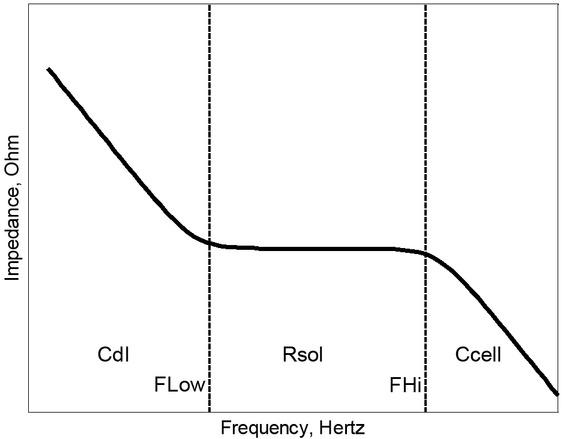Diagram of total impedance vs. frequency behavior. Three cases can be distinguished: For low frequencies (before FLow), the total impedance only depends on the double layer capacitance CDL. In the intermediate range the impedance reaches a plateau, which depends on the solution resistance RSol. For high frequencies (after FHi), the impedance depends on the cell capacitance CCell.

As shown in Figure 1.b, there are two parallel branches (CCell and CDL). When the frequency is not greater than FHi, the current cannot cross the middle of the dielectric capacitor. That is, the capacitor is inactive, and acts as an open circuit. In this case, the total impedance corresponds to the double layer capacitance and solution resistance in series.

Total impedance becomes essentially resistive at frequencies lower than FLow, and CDL it contributes mainly to the total impedance value

$Z≈2+jω⋅CDL⋅RSolj⋅ω⋅CDL$$$\text{Z}\approx \frac{2+\text{j }\!\!\omega\!\!\text{ }\cdot \text{CDL}\cdot \text{RSol}}{\text{j}\cdot \text{ }\!\!\omega\!\!\text{ }\cdot \text{CDL}}$$$FLow≈1π⋅RSol⋅CDL$$$\text{FLow}\approx \frac{1}{\pi \cdot \text{RSol}\cdot \text{CDL}}$$

At lower frequencies, impedance increases with the decrease of the frequency in the double layer region.

However, above FLow, double layer capacitance offers no impedance. This is explained by the fact that only the resistance of the solution contributes to the impedance below FHi when the influence of CCell is not yet significant, thus making total impedance independent of frequency (resistance of the solution zone). This results into a frequency band, restricted by FLow and FHi, in which the results (e.g. the conductivity) can be deduced from the observed impedance:

$Z(jω)=2RLead+RSol$$$\text{Z}\left( \text{j }\!\!\omega\!\!\text{ } \right)=\text{2RLead}+\text{RSol}$$

The measurement sensitivity is higher between FLow and FHi where RSol is dominant. In order to increase the sensitivity, the frequency band should be increased.

The phenomena of double layer (DL) can then be eliminated from the dielectric properties of the electrolyte solution in the diagram of total impedance by shifting the lower cutoff frequency to be as small as possible. Optimizing the impedance cell maximizes the plateau width of the curve in Figure 3.

When the frequency is higher than FHi, the current crosses the middle of the dielectric capacitor instead of crossing the electrolyte solution resistance. That is, the branch containing CDL + RSol + CDL is inactive and the branch with CCell is active. In this zone, the dielectric capacitance of the medium governs the total impedance, and the double layer capacitance and medium resistance can be neglected. Thus, the total impedance value is inversely proportional to the frequency:

$Z≈RSolj⋅ω⋅CCell⋅RSol+1$$$\text{Z}\approx \frac{\text{RSol}}{\text{j}\cdot \text{ }\!\!\omega\!\!\text{ }\cdot \text{CCell}\cdot \text{RSol}+1}$$$fHi≈12⋅π⋅RSol⋅CCell$$$\text{fHi}\approx \frac{1}{2\cdot \text{ }\!\!\pi\!\!\text{ }\cdot \text{RSol}\cdot \text{CCell}}$$

By replacing RSol from equation (1), CDL from equation (4) and CCell from equation (3) respectively, the equations (7) and (10) in terms of conductivity and permittivity parameters become:

$FLow≈σSol0.5⋅π⋅W⋅L⋅N⋅CDL, Surface⋅KCell$$$\text{FLow}\approx \frac{\text{ }\!\!\sigma\!\!\text{ Sol}}{0.5\cdot \text{ }\!\!\pi\!\!\text{ }\cdot \text{W}\cdot \text{L}\cdot \text{N}\cdot \text{CDL,}\,\text{Surface}\cdot \text{KCell}}$$$$\text{FHi}\approx \frac{\text{ }\!\!\sigma\!\!\text{ Sol}}{2\cdot \pi \cdot {{\varepsilon \,}_{0}}\cdot {{\varepsilon \,}_\text{r,}}\,\text{Sol}}$$

Note that the higher boundary frequency, FHi is not dependent on the geometry, according to the theory, when there is no wiring capacitance. Obviously, maximizing the width of the plateau in the diagram of total impedance (Figure 3) can thus only be done by decreasing the lower boundary frequency. In order to make the lower boundary frequency (11) as low as possible, the geometrical term in equation (13) should be maximized.

$W⋅L⋅N⋅KCell (N, L, S, W)$$$\text{W}\cdot \text{L}\cdot \text{N}\cdot \text{KCell}\,\left( \text{N,}\,\,\text{L,}\,\,\text{S,}\,\text{W} \right)$$

We showed in , that the cell constant KCell is inversely proportional to the width of electrodes W. When W increases, KCell decreases and it is impossible to realize the maximization of the geometrical term of equation (13). But when using a square structure of L×L, one variable can be eliminated and the optimization is possible, since L × L : L = N ⋅ (W + S) -S , with L in mm and S in microns:

$L=N⋅(W+S)$$$\text{L}=\text{N}\cdot \left( \text{W}+\text{S} \right)$$

However, it is more illustrative to introduce a factor a = S/W. Using the substitutions:

$W=LN⋅1(a+1) and S=LN⋅a(a+1)$$$\text{W}=\frac{\text{L}}{\text{N}}\cdot \frac{1}{\left( \text{a}+1 \right)}\,\,\text{and}\,\,\text{S}=\frac{\text{L}}{\text{N}}\cdot \frac{\text{a}}{\left( \text{a}+1 \right)}$$

which are based on equation (15) together with the ratio a, expression (13) becomes:

$2.L(N - 1)⋅1(a+1)⋅K(k)K(1-k2)=X (N,L)×Y(a)where X(N,L)=2⋅L(N - 1) and Y(a)=1(a+1)⋅K(k)K(1-k2)$\begin{align}& \,\,\,\,\,\,\,\,\,\frac{2.\text{L}}{\left( \text{N}\,\text{-}\,\text{1} \right)}\cdot \frac{1}{\left( \text{a}+1 \right)}\cdot \frac{\text{K}\left( \text{k} \right)}{\text{K}\left( \sqrt{\text{1-}{{\text{k}}^{2}}} \right)}=\text{X}\,\left( \text{N},\text{L} \right)\times \text{Y}\left( a \right) \\ & \text{where}\,\,\text{X}\left( \text{N},\text{L} \right)=\frac{2\cdot \text{L}}{\left( \text{N}\,\text{-}\,\text{1} \right)}\,\,\text{and}\,\,\text{Y}\left( \text{a} \right)=\frac{1}{\left( \text{a}+1 \right)}\cdot \frac{\text{K}\left( \text{k} \right)}{\text{K}\left( \sqrt{\text{1-}{{\text{k}}^{2}}} \right)} \\ \end{align}

This optimization expression, which has to be maximized in order to minimize FLow, can be split in two parts. The first is the factor X(N, L), showing that the cell size L×L must be as large as possible while reducing the number of fingers. Since there is no maximum in the desired cell size, with respect to the optimization of FLow, the value L can be chosen according to the medium of interest. A cell size of about 1 mm2is used in the modeling. The optimal number of fingers N has a minimum of N = 2 which is the lowest possible number of fingers. On the opposite side, the sensitivity of the impedance measurement depends on the number of fingers.

The sensitivity increases by increasing the area of the contact surface between the electrodes and the sample under test. The modeling allows us to study the influence of the number of fingers on the impedance measurement, showing that a higher number of fingers leads to higher sensitivity. The factor X(N, L) is related to the sum W+S according to equation (14), the second factor in expression (16) has only the ratio W/S = a as a parameter. In Figure 4 this factor is plotted as a function of a varying from 0 to 10. For a = 1, the finger width is equal to the gap between them. It can be seen that this is not the optimal ratio, for a = 0.66 the optimization function has a maximum indicating that the finger width should be approximately 1.5 times as large as the gap width, W = 3S/2. This maximum for the function denoted in the figure as f (a) is equal to 0.66.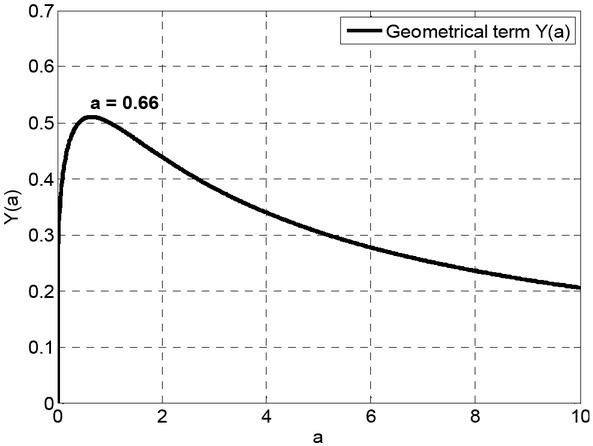Analytical optimization of the dimensional parameters that to say width W and spacing S. The geometrical term Y (a) is a function of S and W, where the ratio a is equal to S/W. Its value of optimization is 0.66.

Therefore, when designing a square structure, the design rule in physical modeling, based on the maximum frequency range criterion according to equation (14) becomes L = (2. 5N − 1) ⋅ S.

Optimization of cell constant

In biological measurements of samples with micrometrical dimensions, it is preferable to use electrode structures with the lowest impedance for the frequency range where the total impedance is equal to the sensing element RSol in order to increase the sensitivity of microbiological measures. Indeed, the impedance variations generated by the cells are more easily detected . And therefore it is preferable to use interdigitated electrodes, which have the minimal cell constant, because RSol depends directly on KCell (equations (1) and (2)).

We analytically studied the variation of the cell constant factor KCell (equation (2)) depending on the number of fingers N (Figure 5), using the first rule of optimization mentioned above, S/W equal 0.66. In this figure we note that the cell constant decreases with the number of fingers N. We can see a wide variation in the cell constant for N ranging from 2 to 20 electrodes. After N reached 20 electrodes the change of KCell became almost negligible.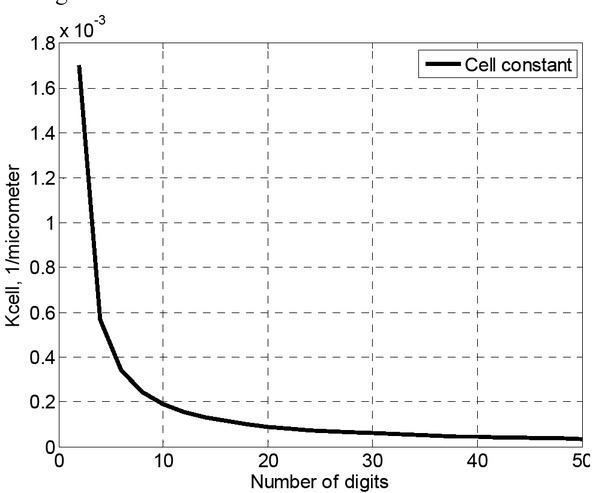The geometrical cell constant KCell by varying the number N of electrodes in the optimization case W = S ⋅ 3/2. The finger length L is 1000 μm.

The two optimization rules have been studied by leaving the surface area of placement of the sample under test L×L constant, but by varying the form factor (cell constant KCell). The first rule of optimization is to choose the electrode width W equal to 1.5 multiplied by the separation between the electrodes S and reduce the number of electrodes as much as possible. The second rule is to increase the number of electrodes to reduce the cell constant as much as possible. As the cell constant does not vary significantly after increasing the number of electrodes to 20, and by superimposing the two optimization rules we chose N equal to 20 as a theoretical optimization of interdigitated electrodes.

3D Modeling system

In this section, the design of the physical model of the sensor loaded by the blood is described. This model was developed for simulation with a finite element method (FEM) using the CoventorWare® software. We used the microelectromechanical systems (MEMS) module for electro quasi-static harmonic response provided by the library of the software and according to this approach. This approach is valid only when the frequency used is less than 107 Hz.

Modeling the electrodes

The structure of the interdigitated impedance sensor used in this simulation is on the order of microns. It is composed of two layers of glass and platinum, layers 1 and 2 respectively (top view shown in Figure 6, 3D view shown in Figure 7). A glass layer of length 1300 μm and width of 1300 μm has been defined as a substrate with thickness of 1000 μm to carry the system (layer 1 on Figure 7). Glass is a good electrical insulator (10-17S⋅m-1) with a relative permittivity (around 5-7 ) that is small. As the glass has a very small permittivity, we do not need to put an insulating layer between the substrate and the electrodes. Next, we defined a mask of platinum electrodes (thickness 1 μm), known to be a good conductor (9.66 ⋅ 106 S·m-1 ), using the graphical editor of CoventorWare. This is deposited on the glass, red layer on Figure 6 and layer 2 on Figure 7. The effective region of electrodes forms a square 1000 μm × 1000 μm and is constant for all different geometries of electrodes.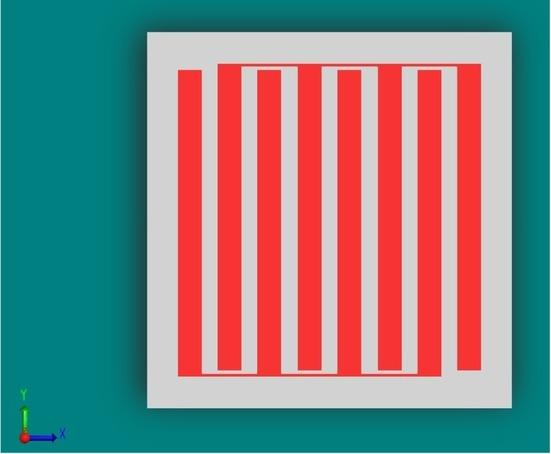Top view of a structural model of 8 electrodes in a planar interdigitated array. The size is 1000×1000 μm, the width W and finger spacing S of the electrodes in this figure are 79 μm and 52 μm, respectively.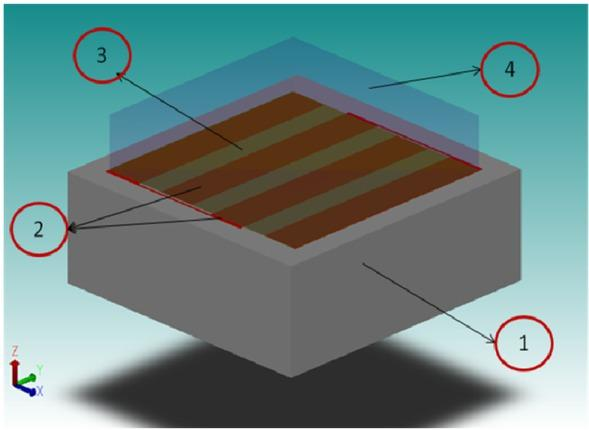3D view of structural model of 4 electrodes type planar interdigitated electrode array (layer 2) loaded by the full medium, which contains the double layer DL (layer 3) and blood medium (layer 4). The entire system is located on a glass substrate (layer 1). The width W and spacing S of electrodes in this figure is 167 μm and 111 μm, respectively. The size of full medium is 1000×1000×500 μm.

The characteristic parameters of the geometry of electrodes, the length of digit L, the number of digits N, the digit width W and the distance between digits S, were selected according to formulas of the first rule of optimization given above. With L fixed at 1000 μm:

$W=3⋅S2μm, S=L(5⋅N/2−1)$$\text{W}=\frac{3\cdot \text{S}}{2}\text{ }\!\!\mu\!\!\text{ m,}\,\,\text{S}=\frac{\text{L}}{\left( 5\cdot {\text{N}}/{2}\;-1 \right)}$ μm and N is a variable.

Then, W, S and N are variable but the surface area of placement of the sample under test is constant because these parameters are used in that way:

$L=N⋅(W+S) - S$$$\text{L}=\text{N}\cdot \left( \text{W}+\text{S} \right)\,\,\text{-}\,\text{S}$$

For example, N=4 electrodes, S=1000/((4×5/2)-1)=111μm and W=111×3/2=167μm.

Modeling the blood medium

From Figures 1 and 3, the components CCell and RSol models the dielectric properties of the medium under testing, and the component CDL models the properties of the double layer interface phenomena. Therefore the total medium is composed of two sub-media. In physical modeling these two sub-media are translated into two layers, one over the other, horizontally on top of the electrodes. On the other hand, in general the type of the equivalent circuit of Figure 1 exempted of the resistances Rlead must be physically modeled in two layers one on top the other in the modeling of CoventorWare according to the references provided by the software package itself . This again verifies our choice of two layers one over the other.

The two layers that describe the interface phenomena occurring at the electrode-electrolyte interface are simplified to a single equivalent layer. The double layer, DL, consists of the interface between platinum electrode and the electrolyte medium, with a thickness of about 5 nm and a relative permittivity about 97 . This is the first layer shown in Figure 7 (layer 3). The second layer is the blood (layer 4).

The total medium is thus composed by two layers. Since the 5 nm thickness creates problems in the computer’s memory for the mesh system, they were shifted to a thickness of 10 μm by scaling (equal to 5 nm × 2 × 1000) which is conformed to the mesh and to a relative permittivity of 194000 (equal to 97 × 2 × 1000) in order to maintain the capacity of the new layer at the same value. One can notice that the DL is mostly an insulator . We tested the model of blood as a biological medium with 0.7 S/m as conductivity in the frequency range 100 – 107  and 80 as relative permittivity at a high frequency around 107 – 108 Hz . Its layer is of 500μm thickness.

Simulation results

The dimensions of the modeled domain are very small at the wavelengths of the electric fields used justifying the quasi-static approximation . The mesh is an essential step in the modeling. An appropriate mesh greatly contributes to optimizing the accuracy of calculation of the system. In the case of our configuration, the physical model mesh was created using a Manhattan mesh with linear elements sized five microns in the X, Y, Z directions (Figure 8).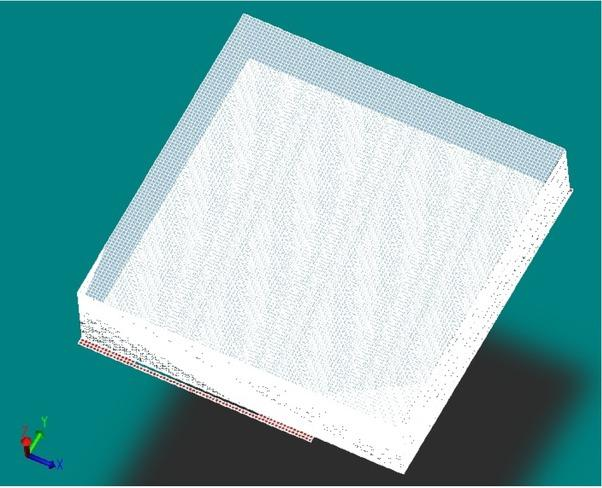3D view of a structural model meshing of 4 electrodes type planar interdigitated array, covered by the full blood medium. The mesh type is Manhattan bricks and the element size is 5 μm.

A 1 volt sinusoidal signal potential between the terminals of the interdigitated electrodes with a frequency range from 100 Hz to 10 MHz was applied. The magnitude of the biological impedance Z was simulated for different cases of electrodes configuration. For the first models, we arbitrarily chose N equal to 16 electrodes.

The magnitude of the electrical impedance Z was calculated from the data capacitance, C, and conductance, G, of the total medium delivered by the software as simulation results (equation (17)). The impedance Z is related to the electrical admittance Y by Z=1/Y.

$1Z=Y = G+jωC$$$\frac{1}{\text{Z}}=\text{Y}\,\text{=}\,\text{G+j}\omega \text{C}$$

Here ω is the frequency and j is the imaginary number.

To verify the structure of the physical model, chosen as a full medium composed of two layers, DL, and blood medium, we observed the influence of the double layer interface on the simulated electrical impedance of the full medium by comparing simulation results of two system models with and without the DL. Figure 9 shows the sensor response of 16 electrodes for these two cases. One can see the response of the first case that with DL corresponds to the schematic diagram of the total impedance appeared in Figure 3, but the phenomena of the dielectric capacity CCell of the blood medium do not appear because the cut off frequency FHi is higher than 107 Hertz. For the second case without DL, one can notice that the impedance is constant throughout the frequency range and does not take into account the cut-off frequencies FLow.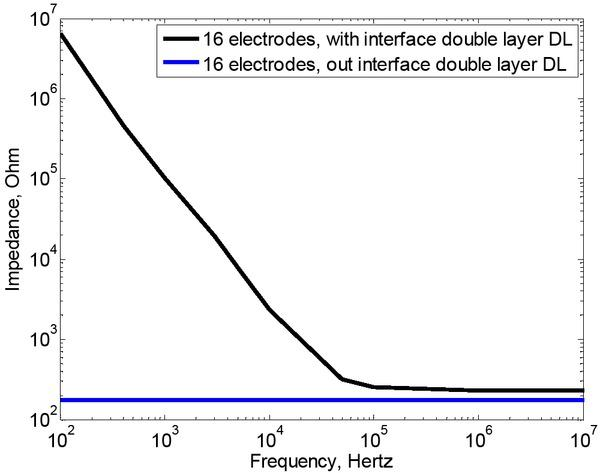Simulated electrical impedance for a full blood medium deposited on the interdigitated structure of 16 electrodes. The medium is modeled with and without interface double layer DL.

In the purpose of verifying the choice of values (5200) of the dielectric properties of blood, in particular the permittivity, we studied the influence of changing its value on simulated impedance response of the interdigital sensor with 16 electrodes, displayed in Figure 10. By comparing simulation results for two different permittivities (80 and 5200, respectively), one can observe that the permittivity does not affect the impedance reaction for a frequency range between 100 and 107 Hertz.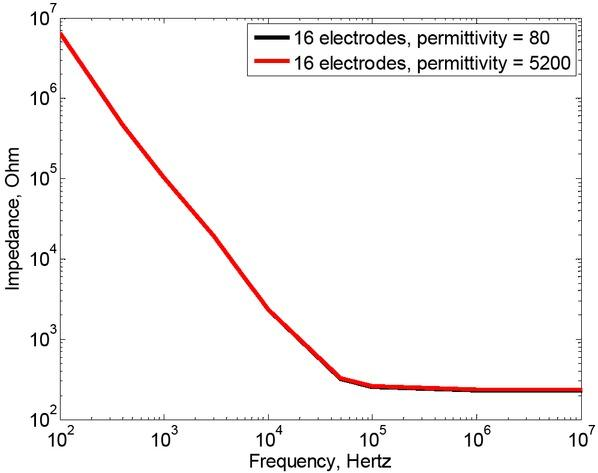Simulated response of interdigital biosensor with 16 electrodes, by changing the permittivity of the blood medium (layer 4). Electrical impedance simulated for two different permittivities, 80 and 5200, respectively.

Figure 11 shows the influence of changing the conductivity of the blood medium. Simulation results are obtained for two different conductivities, 0.7 and 9 S/m, respectively. When the conductivity increases, the RSol decreases then the plateau shifts to lower impedance. In addition, a change in the height of the plateau implies a change in the boundary frequency FLow.Simulated response of interdigital biosensor with 16 electrodes, by changing the conductivity of the blood medium (layer 4). Electrical impedance simulated for two different conductivities, 0.7 S/m and 9 S/m, respectively.

A main objective is the verification of the results of theoretical development of equations linking the design parameters and the behavior of frequency for coplanar impedance sensors, in the first rule of optimization by S/W equal 0.66. In the Figure 12 we showed the influence of the ratio between the width of electrodes and the separation between electrodes. Figure 12a shows the simulated electrical impedance in three different cases of S/W where this factor equal 0.66, 0.1 and 5. To be able to simulate these three cases of S/W, we had to develop systems with eight electrodes. In this figure the cut off frequencies amongst the different types seem very close. Thus, to distinguish the three cut off frequencies for each geometry Figure 12b presents the phase curves corresponding to each case.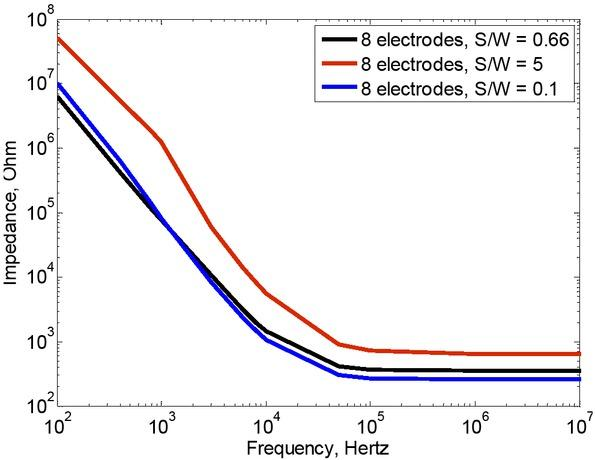Behavior of simulated electrical impedance for the blood medium by varying the cell constant of the sensor with changing the ratio between width of electrodes and gap, while leaving the same contact area of sensor 1000 × 1000 microns by N = 8 electrodes.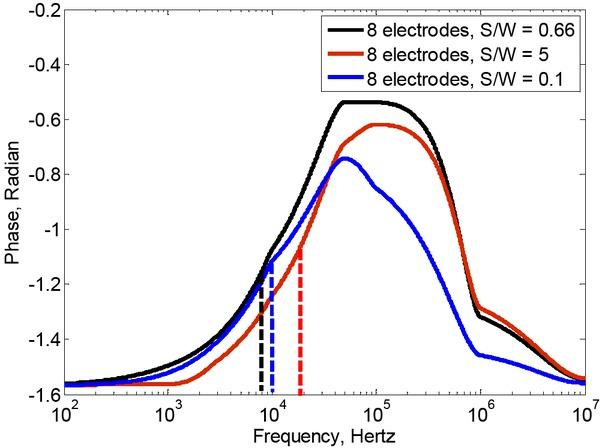Behavior of corresponding electrical phase by varying the ratio between width of electrodes and gap.

To confirm the second rule of optimization Figure 13 gives simulation results of change in electrical impedance response and electrical phase for many sensors with different electrodes values N. Using the conditions of the first rule of sensor optimization mentioned above, that spacing over width of electrode S/W equal 0.66, six different structures were developed and modeled with respect that the surface area of placement of the full medium under test is constant for all sensors structure. In Figure 13a we study the change in impedance plateau values RSol and the cutoff frequency FLow are given by figure 13b for different numbers of electrodes (4, 8, 12, ...) and it is compared to the case N = 2. This case is taken as reference since this is the lowest possible number of fingers. So we study the sensitivity of the sensors relative to case 2 electrodes. In this case, we can see that the impedance shows a plateau for frequencies above FLow = 3·103 Hz. This level is 2000 Ω. For 4 electrodes, one can see a different frequency FLow equal to 5·103 Hz and the plateau is much changed compared to the case 2 electrodes, and this change is 700 Ω. For N equals 8, one can see a different frequency FLow equal 7·103 Hz and the plateau dominated by RSol is changed compared to the case with 4 electrodes, and the change is 350 Ω. When N is equal to 12, the platform decreases slightly compared to the case with 8 electrodes with a value of 260 Ω and FLow = 1·104 Hz. The curves of 16 and 20 electrodes have almost same value of the plateau 220 Ω with a low cut-off frequency 1.6·104 and 1.7·104 Hz respectively.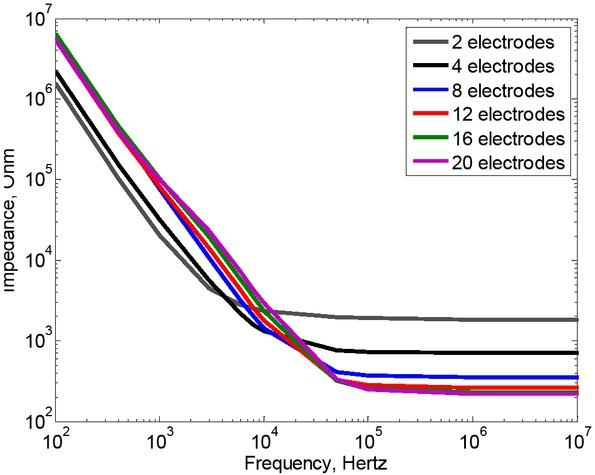Behavior of simulated electrical Bioimpedance for the blood medium by varying the cell constant of the sensor with changing the number of electrodes N, while leaving the same contact area of sensor 1000 × 1000 microns.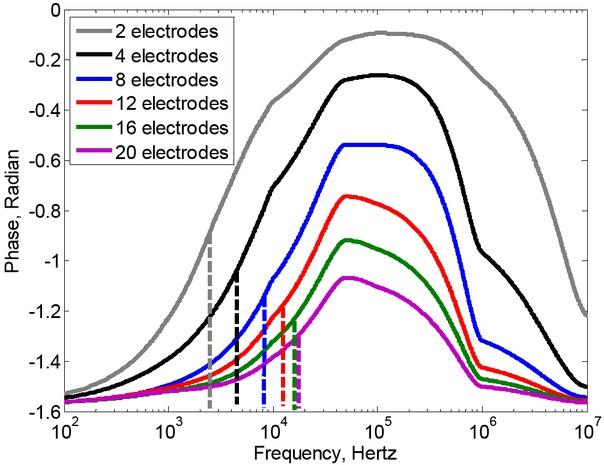Behavior of corresponding electrical phase by varying the number of electrodes N.

As we mentioned in the paragraph of Optimizing the electrodes geometry, the optimal number of fingers N has a minimum for N = 2 which is the lowest possible number of fingers. Conversely, the sensitivity of the impedance measurement depends on the number of fingers.

The sensitivity increases by increasing the contact surface between the electrodes and the sample under test . Then, the modeling allows us to study the influence of the number of fingers on the measurement sensitivity. In order to fully exploit the variation of sensors impedance depending on the number of electrodes, N, the impedance difference related to the number of electrodes has been investigated in Figure 14. We study the sensitivity of detection i.e. the change in the sensor’s impedance response for the same numbers of electrodes mentioned in Figure 13, in order to compare it to the N = 2 case that is the optimal number relative to the optimization of frequency range but with the smallest measurement sensitivity. We introduce a calculation for the sensitivity as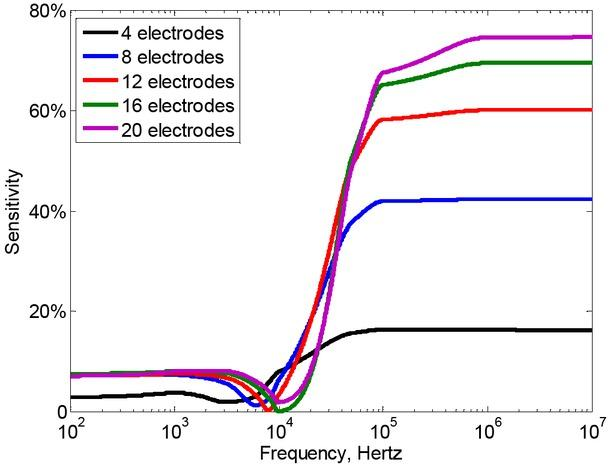Difference sensitivity percentage of simulated electrical Bioimpedance for the blood medium relative to the 2 electrodes type sensor by varying the number of electrodes N, while leaving the same contact area of sensor 1000 × 1000 microns. The sensitivity is calculated by (Zi-Z2)/ (Z2·volume), i is the number of electrodes.$(Zi−Z2)(Z2⋅Volume)$$$\frac{\left( \text{Zi}-\text{Z2} \right)}{\left( \text{Z}2\cdot \text{Volume} \right)}$$

where i is the number of electrodes.

We can note that this change in impedance for the 2 electrode configuration is very small below the frequency FLow (<10%), and presents good values for frequencies greater than FLow. For 4 electrodes the sensitivity was 16 %. For 8 electrodes the sensitivity was 42 %. For 12 electrodes the sensitivity was. 58 %. For 16 electrodes the sensitivity was 68 %. In arriving at 20 electrodes the sensitivity increases only 4 % compared to 16 electrodes.

Discussion

As can be seen in the figures showing the response of the impedance and phase, when the impedance is constant as a function of the frequency corresponding to RSol, the proper phase angle cannot reach zero degrees because the impedance is not entirely completely resistive. There is always the capacitive effect of the medium.

By comparing the two curves in Figure 9, the effects of the capacity of double layer interface CDL on the curve of the impedance simulated do not exist for the physical model without the presence of the defined layer DL (layer 3 in Figure 7). The conduction currents passing through RSol are subject to double-layer phenomena because they propagate through in the sample via CDL. The absence of the layer DL in the physical model of the full medium, translates into the impedance consists of only resistance RSol in parallel with capacitance CCell that has a negligible effect for low frequencies, which is suitable for the fact that the value of CDL, in the equivalent circuit model of Figure 1, tends toward infinity, causing the value of the cut off frequency FLow in the equation 11 to tend toward zero, i.e the impedance curve remains constant over the entire frequency range. Therefore the choice of two layers DL and blood medium to make the blood full medium is warranted.

Both simulated curves for two different permittivities in Figure 10 are confounded in the low frequency range. The permittivity does not affect the impedance for frequencies less than 107 Hertz. Thus the effects of capacity CCell representing the dielectric properties of the medium does not appear only for high frequencies. Both curves have the same low cut off frequency FLow. This justifies the value of FLow in equation (11) that does not depend on the permittivity of the medium.

The change of the medium’s conductivity in Figure 11 changes the level of the plateau of RSol and changes the FLow confirming the validation of the two equations (2) and (11) of RSol and FLow, respectively.

In Figure 13 it was shown that the RSol element is sensitive because the sensitivity compared to the 2 electrodes case does not have good values below FLow.

The three cut off frequencies found from Figure 12 are 7·103, 10·103 and 20·103 corresponding to W/S = 0.66, 0.1 and 5, respectively. Table 1 is more representative and shows the difference percentage between the case and the standard 0.66. In the case of 0.1, there is a difference percentage of 43% and in the case 5 there is a percentage of 186%. These values (43%, 186%) are relatively large compared to case 0.66 and verify the theory of the first rule of optimization. Furthermore the difference percentage in the case 0.1 is relatively low compared to the case 5. This is in agreement with the curve Y(a) in Figure 4. We must note that for very low conductivities, the change in flow caused by changing the geometry is more important.

Representative table of the variation of cut off frequency FLow depending on the geometry.

FLow (Hz)ΔFLow (Hz)change Relative %
S/W = 0.667·10300
S/W = 0.110·1033·10343
S/W = 520·10313·103186

The influence of the geometry of a sensing system with interdigital electrodes; the width, the separation and the number of electrodes on the detected impedance in the medium was validated (Figure 13). Indeed, the increasing number of electrodes increases the low cut off frequency, and a diminution in the impedance into the intermediate frequency range where RSol dominates. It verifies the dependence of parameters FLow and RSol by the geometry of electrodes in equations (2), (11), (13) and (16). It was observed that the change in the simulated impedance response by varying the number of electrodes is not significantly detected by passing 16-20 electrodes. This is consistent with Figure 5.

According to the curves of the impedance in Figure 13a we cannot see the high cut off frequency, but according to curves of the phase in Figure 13b one finds a common cut off for all geometries at approximately 106 Hz. This can be explained in two ways: The first is that we really have a capacitive effect about 106 Hz, but it is not pure and that the values of the G are very large compared to the values of C, so we cannot find this frequency in the curve impedance. The second way is that as we mentioned on the validity of the model above 107 Hz, there was doubt for these values at high frequency around 106 – 107 Hz.

The change in impedance resulting from variation in the number of electrodes relative to the impedance of two electrodes presented in Figure 14 shows that these measurement systems are sensitive to frequencies greater than FLow. For the frequencies less than FLow, the sensitivity is very low. It justifies why we tried to shift the FLow to minimum. It can be noted also that the cut off frequencies did not change by varying N, especially for the last three cases (12, 16 and 20 electrodes). The variations in the impedance dominated by RSol are negligible above 20 electrodes, which is consistent with Figure 5. This sensitivity of detection makes further proof that the sensing element of measures is the RSol, listed in the electrical circuit of Figure 1.

Taking into consideration the two optimization rules we have presented, it can be confirmed based on the analytic geometry optimization of the sensor and according to the simulated impedance in the section physical modeling of the full system, the optimized geometry of an interdigital sensor with size 1mm×1mm, is suitable for 20 electrodes having a width 20 microns and spacing 30 microns confirming the first rule of optimization. Generally the optimized geometry of an interdigital sensor is to choose a ratio between the gap and the width of electrodes equal 0.66.

The results of the theoretical development of optimization of sensor and those of the 3D modeling and simulation are consistent.

Conclusion

This paper presents a comparative approach for simulation of an interdigitated sensor in a biological medium using CoventorWare® software. Three dimensional interdigital sensor simulation techniques were done to analyze the influence of the physical properties of the medium and the impedance response by optimizing the geometry of sensor was used. This optimization method used for bioimpedance spectroscopy sensor was obtained from theoretical equations, by developing total impedance equations and modeling equivalent circuits. Sensitivity related to the number of electrodes has been studied and a geometrical structure of the sensor was optimized. The simulation results are in agreement with the theoretical equations of optimization. We found that the optimized geometry of an interdigital sensor is to choose a ratio between the gap and the width of electrodes equal 0.66 to expand the useful frequency band. It is thus possible to design a fully optimized interdigitated sensor by including a priori the biological medium of interest that will load it.

#### Representative table of the variation of cut off frequency FLow depending on the geometry.

FLow (Hz)ΔFLow (Hz)change Relative %
S/W = 0.667·10300
S/W = 0.110·1033·10343
S/W = 520·10313·103186

Grimnes S, Martinsen OG. Bioimpedance and Bioelectricity Basics. Academic Press; 2000.GrimnesSMartinsenOGBioimpedance and Bioelectricity BasicsAcademic Press200010.1016/B978-012303260-7/50009-5Search in Google Scholar

Faes TJ, Meij HA, De Munck JC, Heethaar RM. The electric resistivity of human tissues (100 Hz-10 MHz): a meta-analysis of review studies. Physiol Meas 1999, 20:R1-10. http://dx.doi.org/10.1088/0967-3334/20/4/2011059322610.1088/0967-3334/20/4/201FaesTJMeijHADeMunck JCHeethaarRMThe electric resistivity of human tissues (100 Hz-10 MHz): a meta-analysis of review studiesPhysiol Meas199920R110http://dx.doi.org/10.1088/0967-3334/20/4/201Search in Google Scholar

Katz E, Willner I. Probing Biomolecular Interactions at Conductive and Semiconductive Surfaces by Impedance Spectroscopy: Routes to Impedimetric Immunosensors, DNA-Sensors, and Enzyme Biosensors. Electroanalysis, 15 (11), 913-947, 2003. http://dx.doi.org/10.1002/elan.20039011410.1002/elan.200390114KatzEWillnerIProbing Biomolecular Interactions at Conductive and Semiconductive Surfaces by Impedance Spectroscopy: Routes to Impedimetric Immunosensors, DNA-Sensors, and Enzyme BiosensorsElectroanalysis15119139472003http://dx.doi.org/10.1002/elan.200390114

Sheppard NF, Tucker RC, Wu C. Electrical-Conductivity Measurements Using Microfabricated Interdigitated Electrodes. Analytical Chemistry, 1993, 65(9), p. 1199-1202. http://dx.doi.org/10.1021/ac00057a01610.1021/ac00057a016SheppardNFTuckerRCWuCElectrical-Conductivity Measurements Using Microfabricated Interdigitated ElectrodesAnalytical Chemistry199365911991202http://dx.doi.org/10.1021/ac00057a016

Mukhopadhyay SC. Sensing and Instrumentation for a Low Cost Intelligent Sensing System. SICE-ICASE International Joint Conference, Bexco, Busan, Korea, pp 1075-1080, Oct 2006. http://dx.doi.org/10.1109/SICE.2006.315815MukhopadhyaySCSensing and Instrumentation for a Low Cost Intelligent Sensing SystemSICE-ICASE International Joint Conference, Bexco, Busan, Korea107510802006http://dx.doi.org/10.1109/SICE.2006.31581510.1109/SICE.2006.315815Search in Google Scholar

Mukhopadhyay SC, Gooneratne CP, Demidenko S, Sen Gupta G. Low cost sensing system for dairy products quality monitoring. Proceedings of 2005 International Instrumentation and Measurement Technology Conference, Ottawa, Ont 2005, IEEE Catalog Number 05CH37627C, ISBN 0-7803-8880-1, 244-249.MukhopadhyaySCGooneratneCPDemidenkoSSenGupta GLow cost sensing system for dairy products quality monitoring. Proceedings of 2005 International Instrumentation and Measurement Technology ConferenceOttawaOnt 2005, IEEE Catalog Number 05CH37627C, ISBN 0-7803-8880-1244249Search in Google Scholar

Van Gerwen P, Laureyn W, Laureys W, Huyberechts G, Op De Beeck M, Baert K, Suls F, Sansen W, Jacobs P, Hermans L, Mertens R. Nanoscaled interdigitated electrode arrays for biochemical sensors. Sens. Actuators, B 49.1999.73–80.VanGerwen PLaureynWLaureysWHuyberechtsGOpDe Beeck MBaertKSulsFSansenWJacobsPHermansLMertensRNanoscaled interdigitated electrode arrays for biochemical sensorsSens. ActuatorsB 49.1999738010.1016/S0925-4005(98)00128-2Search in Google Scholar

Timms S, Colquhoun KO, Fricker CR. Detection of Escherichia coli in potable water using indirect impedance technology. J. Microbiol, Meth, 26(1996) 125. http://dx.doi.org/10.1016/0167-7012(96)00903-710.1016/0167-7012(96)00903-7TimmsSColquhounKOFrickerCRDetection of Escherichia coli in potable water using indirect impedance technologyJ. Microbiol, Meth261996125http://dx.doi.org/10.1016/0167-7012(96)00903-7

Geng P, Zhang X, Meng W, Wang Q, Jin L, Feng Z, Wu Z. Self-assembled monolayers-based immunosensor for detection of Escherichia coli using electrochemical impedance spectroscopy. Electrochim, Acta 53 (2008) 4663. http://dx.doi.org/10.1016/j.electacta.2008.01.03710.1016/j.electacta.2008.01.037GengPZhangXMengWWangQJinLFengZWuZSelf-assembled monolayers-based immunosensor for detection of Escherichia coli using electrochemical impedance spectroscopyElectrochimActa5320084663http://dx.doi.org/10.1016/j.electacta.2008.01.037

Franks W, Schenker I, Schmutz P, Hierlemann A, Impedance characterization and modeling of electrodes for biomedical applications. 2005. IEEE Trans. Biomed. Eng. 52, 1295–1302. http://dx.doi.org/10.1109/TBME.2005.8475231604199310.1109/TBME.2005.847523FranksWSchenkerISchmutzPHierlemannAImpedance characterization and modeling of electrodes for biomedical applications. 2005IEEE Trans. Biomed. Eng5212951302http://dx.doi.org/10.1109/TBME.2005.84752316041993Search in Google Scholar

Hong J, Yoon DS, Kim SK, Kim TS, Kim S, Pak EY, et al. AC frequency characteristics of coplanar impedance sensors as design parameters. Lab Chip. 2005;5(3):270-279. http://dx.doi.org/10.1039/b410325d10.1039/b410325d15726203HongJYoonDSKimSKKimTSKimSPakEYet alAC frequency characteristics of coplanar impedance sensors as design parametersLab Chip200553270279http://dx.doi.org/10.1039/b410325d15726203

Linderholm P, Bertsch A, Renaud P. Resistivity probing of multi-layered tissue phantoms using microelectrodes. Physiol Meas. 2004 Jun; 25(3):645-658. http://dx.doi.org/10.1088/0967-3334/25/3/0051525311610.1088/0967-3334/25/3/005LinderholmPBertschARenaudPResistivity probing of multi-layered tissue phantoms using microelectrodesPhysiol Meas2004Jun;253645658http://dx.doi.org/10.1088/0967-3334/25/3/005Search in Google Scholar

Olthuis W et al. Theoretical and experimental determination of cell constants of planar-interdigitated electrolyte conductivity sensors. Sensors and Actuators B 24-25 (1995) 252-256. http://dx.doi.org/10.1016/0925-4005(95)85053-8OlthuisWet alTheoretical and experimental determination of cell constants of planar-interdigitated electrolyte conductivity sensorsSensors and Actuators B 24-251995252256http://dx.doi.org/10.1016/0925-4005(95)85053-810.1016/0925-4005(95)85053-8Search in Google Scholar

Igreja R, Dias CJ. Analytical evaluation of the inter-digital electrodes capacitance for a multi-layered structure. Sensors and actuators A 112 (2004) 291-301. http://dx.doi.org/10.1016/j.sna.2004.01.04010.1016/j.sna.2004.01.040IgrejaRDiasCJAnalytical evaluation of the inter-digital electrodes capacitance for a multi-layered structureSensors and actuators A1122004291301http://dx.doi.org/10.1016/j.sna.2004.01.040

Grimnes S, Martinsen OG. Bioimpedance and bioelectricity basics. 2nd ed. Academic; 2008.GrimnesSMartinsenOGBioimpedance and bioelectricity basics2nd ed. Academic;200810.1016/B978-0-12-374004-5.00010-6Search in Google Scholar

Bard AJ, Faulkner LR. Electrochemical methods, fundamentals and applications, John Wiley and Sons, New York, 1980.BardAJFaulknerLRElectrochemical methods, fundamentals and applicationsJohn Wiley and SonsNew York1980Search in Google Scholar

Ibrahim M, Kourtiche D, Nadi M. The influence of interdigitated electrode configuration modeling for the bioimpedance of biological medium. Proceedings of 2010 4th International Conference on Sensing Technology Lecce, Italia, pp. 578-581.IbrahimMKourticheDNadiMThe influence of interdigitated electrode configuration modeling for the bioimpedance of biological medium. Proceedings of 2010 4th International Conference on Sensing Technology LecceItalia578581Search in Google Scholar

Timmer B, Sparreboom W, Olthuis W, Bergveld P, Berg AVD. Optimization of an electrolyte conductivity detector for measuring low ion concentrations. Lab Chip. 2002;2(2):121-124. http://dx.doi.org/10.1039/b201225a10.1039/b201225a15100845TimmerBSparreboomWOlthuisWBergveldPBergAVDOptimization of an electrolyte conductivity detector for measuring low ion concentrationsLab Chip200222121124http://dx.doi.org/10.1039/b201225a15100845

CRM, Formulaires et tables, ed. Tricorne. 2000.CRM, Formulaires et tables, edTricorne2000Search in Google Scholar

CoventoreWare® Analyzer version 2010. Reference MEMS and Microsystems Design, section MemElectro. www.coventor.comCoventoreWare® Analyzer version 2010. Reference MEMS and Microsystems Design, section MemElectrowww.coventor.comSearch in Google Scholar

Bard AJ, Faulkner LR. Electrochemical Methods, New York, Willey, 2001.BardAJFaulknerLRElectrochemical MethodsNew York, Willey2001Search in Google Scholar

Dielectric Properties of Body Tissues in the frequency range 10Hz-100GHz. www.niremf.ifac.cnr.it/tissprop/htmlclie/htmlclie.htmDielectric Properties of Body Tissues in the frequency range 10Hz-100GHzwww.niremf.ifac.cnr.it/tissprop/htmlclie/htmlclie.htmSearch in Google Scholar

Jaspard F, Nadi M, Rouane A. Dielectric properties of blood : an investigation of haematocrit dependance, Physiological Measurement, Vol 24 pages 134-147, 2003. http://dx.doi.org/10.1088/0967-3334/24/1/310JaspardFNadiMRouaneADielectric properties of blood : an investigation of haematocrit dependance, Physiological MeasurementVol 241341472003http://dx.doi.org/10.1088/0967-3334/24/1/31010.1088/0967-3334/24/1/31012636192Search in Google Scholar

Zimmerman WBJ. Multiphysics Modeling With Finite Element Methods (Series on Stability, Vibration and Control of Systems, Serie). World Scientific Publishing Co., Inc. River Edge, NJ, USA, 2006.ZimmermanWBJMultiphysics Modeling With Finite Element Methods (Series on Stability, Vibration and Control of Systems, Serie)World Scientific Publishing Co., Inc. River Edge, NJ, USA200610.1142/6141Search in Google Scholar

Alexander Frank Jr, Price Dorielle T, Bhansali, Shekhar, Optimization of Interdigitated Electrode (IDE) Arrays for Impedance Based Evaluation of Hs 578T Cancer Cells, J. Phys.: Conf. Ser. 224 012134, Volume 224, Issue 1, pp. 012134 (2010).AlexanderFrank JrPriceDorielle TBhansali, Shekhar, Optimization of Interdigitated Electrode (IDE) Arrays for Impedance Based Evaluation of Hs 578T Cancer CellsJ. PhysConf. Ser. 224 012134Volume 224Issue 10121342010Search in Google Scholar

Empfohlene Artikel von Trend MD